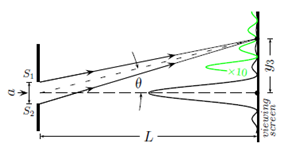# Problem: Consider the setup of a single slit experiment. Determine the height y3, where the third minimum occurs. 1. y3 = 5λ/a L 2.  y3 = 7λ/2a L 3.  y3 = λ/a L 4.  y3 = 3λ/2a L 5.  y3 = 9λ/2a L 6.  y3 = 2λ/a L 7.  y3 = 4λ/a L 8.  y3 = 5λ/2a L 9.  y3 = 3λ/a L 10.  y3 = λ/2a L

###### FREE Expert Solution
86% (407 ratings)
###### Problem Details

Consider the setup of a single slit experiment. Determine the height y3, where the third minimum occurs.

1. y= 5λ/a L

2.  y= 7λ/2a L

3.  y= λ/a L

4.  y= 3λ/2a L

5.  y= 9λ/2a L

6.  y= 2λ/a L

7.  y= 4λ/a L

8.  y= 5λ/2a L

9.  y= 3λ/a L

10.  y= λ/2a LFrequently Asked Questions

What scientific concept do you need to know in order to solve this problem?

Our tutors have indicated that to solve this problem you will need to apply the Single Slit Diffraction concept. You can view video lessons to learn Single Slit Diffraction. Or if you need more Single Slit Diffraction practice, you can also practice Single Slit Diffraction practice problems.

How long does this problem take to solve?

Our expert Physics tutor, Juan took 3 minutes and 11 seconds to solve this problem. You can follow their steps in the video explanation above.